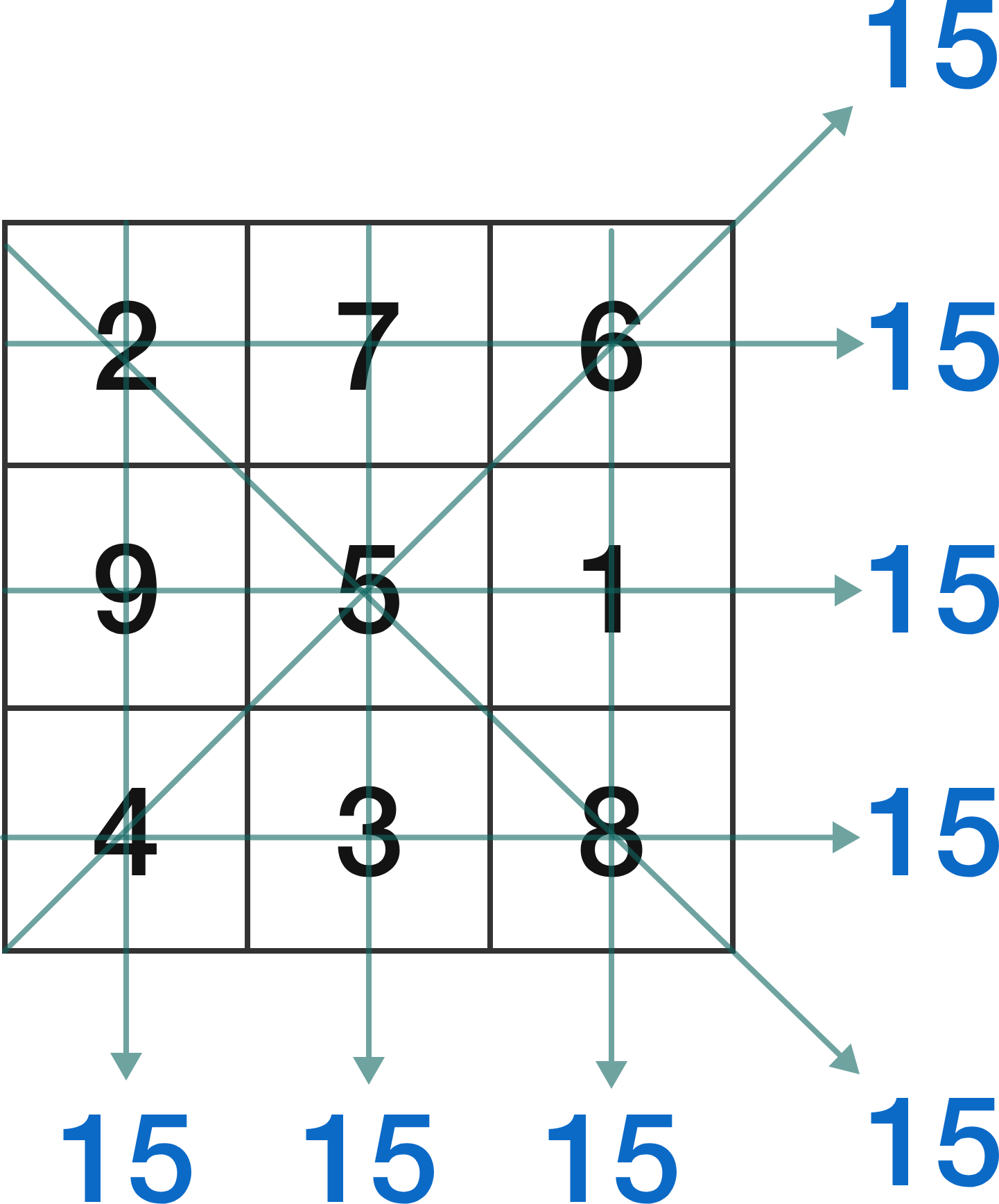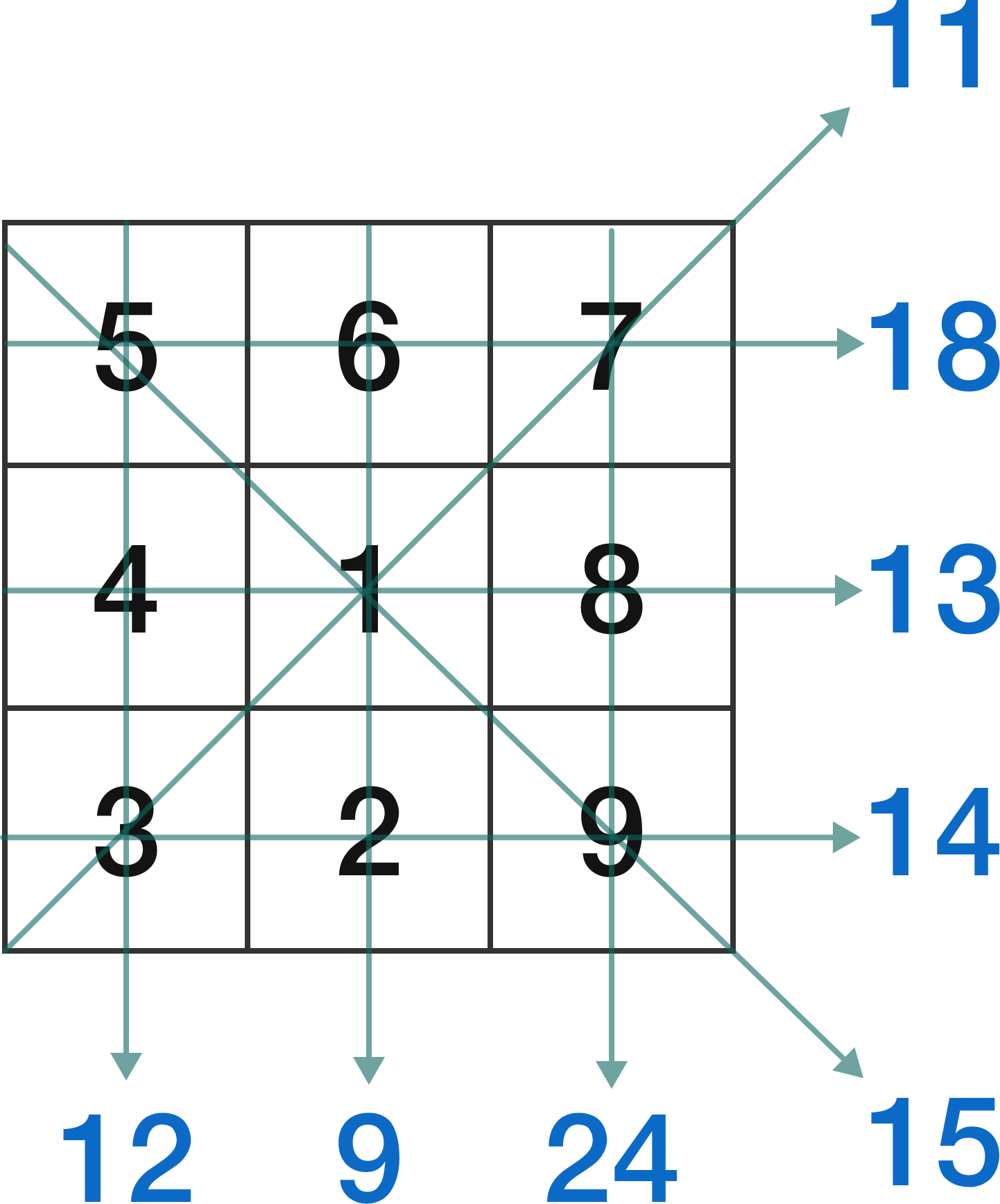# Open Problem #2 test

A magic square contains positive consecutive integers starting from 1 such that the rows, columns, and diagonals all add to the same value.A heterosquare contains positive consecutive integers starting from 1 such that the rows, columns, and diagonals all add to different values.If the sums resulting from a heterosquare form a consecutive sequence, the heterosquare is also called an antimagic square. (In the example above, if the sums of 9 and 24 were 16 and 17 instead, it would be a antimagic square.)

Prove that there are no 3 by 3 antimagic squares.

Unlike with Open Problem #1, this has been proven by computer brute force. The task here is to prove the result by something other than brute force.

For the second Open Problem I wanted to emphasize the fact that mathematics doesn't just emphasize unknown pieces of knowledge; it also improves existing knowledge. A brute force proof doesn't give much insight into the nature of a mathematical structure, so a more elegant proof of the lack of 3 by 3 antimagic squares would be quite helpful for mathematicians working on the subject.

A related (but likely more difficult) open problem is: does an $n$ by $n$ antimagic square exist for all $n > 3 ?$ The answer is thought to be "yes" but nobody knows for sure.

Since heterosquares are a superset of antimagic squares, one approach to this would be finding a systematic way of categorizing all heterosquares, and then looking for the special properties heterosquares that are not antimagic have.

It's also possible to approach from the brute force computing end (even thought it's been done before) and work on ways of gradually improving the algorithm for identifying antimagic squares.

THERE IS A WIKI PAGE DEDICATED TO THE PROBLEM HERENote by Unsuk Chin
3 years, 6 months ago

This discussion board is a place to discuss our Daily Challenges and the math and science related to those challenges. Explanations are more than just a solution — they should explain the steps and thinking strategies that you used to obtain the solution. Comments should further the discussion of math and science.

When posting on Brilliant:

• Use the emojis to react to an explanation, whether you're congratulating a job well done , or just really confused .
• Ask specific questions about the challenge or the steps in somebody's explanation. Well-posed questions can add a lot to the discussion, but posting "I don't understand!" doesn't help anyone.
• Try to contribute something new to the discussion, whether it is an extension, generalization or other idea related to the challenge.
• Stay on topic — we're all here to learn more about math and science, not to hear about your favorite get-rich-quick scheme or current world events.

MarkdownAppears as
*italics* or _italics_ italics
**bold** or __bold__ bold
- bulleted- list
• bulleted
• list
1. numbered2. list
1. numbered
2. list
Note: you must add a full line of space before and after lists for them to show up correctly
paragraph 1paragraph 2

paragraph 1

paragraph 2

[example link](https://brilliant.org)example link
> This is a quote
This is a quote
    # I indented these lines
# 4 spaces, and now they show
# up as a code block.

print "hello world"
# I indented these lines
# 4 spaces, and now they show
# up as a code block.

print "hello world"
MathAppears as
Remember to wrap math in $$ ... $$ or $ ... $ to ensure proper formatting.
2 \times 3 $2 \times 3$
2^{34} $2^{34}$
a_{i-1} $a_{i-1}$
\frac{2}{3} $\frac{2}{3}$
\sqrt{2} $\sqrt{2}$
\sum_{i=1}^3 $\sum_{i=1}^3$
\sin \theta $\sin \theta$
\boxed{123} $\boxed{123}$

## Comments

There are no comments in this discussion.

×

Problem Loading...

Note Loading...

Set Loading...